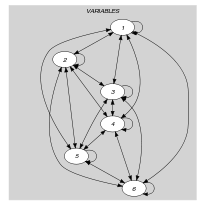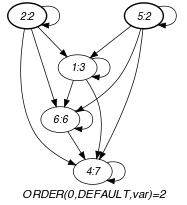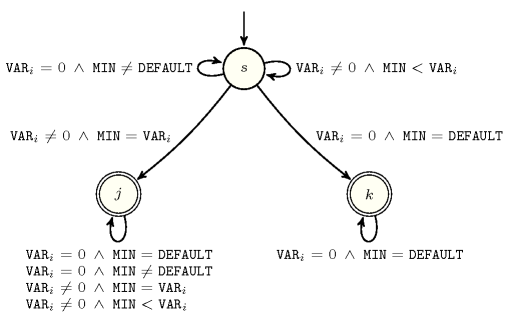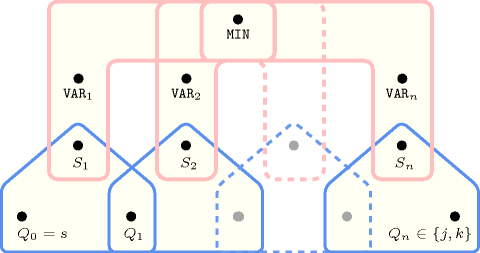## 5.263. minimum_except_0

Origin
Constraint

$\mathrm{𝚖𝚒𝚗𝚒𝚖𝚞𝚖}_\mathrm{𝚎𝚡𝚌𝚎𝚙𝚝}_\mathtt{0}\left(\mathrm{𝙼𝙸𝙽},\mathrm{𝚅𝙰𝚁𝙸𝙰𝙱𝙻𝙴𝚂},\mathrm{𝙳𝙴𝙵𝙰𝚄𝙻𝚃}\right)$

Arguments
 $\mathrm{𝙼𝙸𝙽}$ $\mathrm{𝚍𝚟𝚊𝚛}$ $\mathrm{𝚅𝙰𝚁𝙸𝙰𝙱𝙻𝙴𝚂}$ $\mathrm{𝚌𝚘𝚕𝚕𝚎𝚌𝚝𝚒𝚘𝚗}\left(\mathrm{𝚟𝚊𝚛}-\mathrm{𝚍𝚟𝚊𝚛}\right)$ $\mathrm{𝙳𝙴𝙵𝙰𝚄𝙻𝚃}$ $\mathrm{𝚒𝚗𝚝}$
Restrictions
 $\mathrm{𝙼𝙸𝙽}>0$ $\mathrm{𝙼𝙸𝙽}\le \mathrm{𝙳𝙴𝙵𝙰𝚄𝙻𝚃}$ $|\mathrm{𝚅𝙰𝚁𝙸𝙰𝙱𝙻𝙴𝚂}|>0$ $\mathrm{𝚛𝚎𝚚𝚞𝚒𝚛𝚎𝚍}$$\left(\mathrm{𝚅𝙰𝚁𝙸𝙰𝙱𝙻𝙴𝚂},\mathrm{𝚟𝚊𝚛}\right)$ $\mathrm{𝚅𝙰𝚁𝙸𝙰𝙱𝙻𝙴𝚂}.\mathrm{𝚟𝚊𝚛}\ge 0$ $\mathrm{𝚅𝙰𝚁𝙸𝙰𝙱𝙻𝙴𝚂}.\mathrm{𝚟𝚊𝚛}\le \mathrm{𝙳𝙴𝙵𝙰𝚄𝙻𝚃}$ $\mathrm{𝙳𝙴𝙵𝙰𝚄𝙻𝚃}>0$
Purpose

All variables of the collection $\mathrm{𝚅𝙰𝚁𝙸𝙰𝙱𝙻𝙴𝚂}$ are assigned a value that belongs to interval $\left[0,\mathrm{𝙳𝙴𝙵𝙰𝚄𝙻𝚃}\right]$. $\mathrm{𝙼𝙸𝙽}$ is the minimum value of the collection of domain variables $\mathrm{𝚅𝙰𝚁𝙸𝙰𝙱𝙻𝙴𝚂}$, ignoring all variables that take 0 as value. When all variables of the collection $\mathrm{𝚅𝙰𝚁𝙸𝙰𝙱𝙻𝙴𝚂}$ are assigned value 0, $\mathrm{𝙼𝙸𝙽}$ is set to the default value $\mathrm{𝙳𝙴𝙵𝙰𝚄𝙻𝚃}$.

Example
 $\left(3,〈3,7,6,7,4,7〉,1000000\right)$ $\left(2,〈3,2,0,7,2,6〉,1000000\right)$ $\left(1000000,〈0,0,0,0,0,0〉,1000000\right)$

The three examples of the $\mathrm{𝚖𝚒𝚗𝚒𝚖𝚞𝚖}_\mathrm{𝚎𝚡𝚌𝚎𝚙𝚝}_\mathtt{0}$ constraint respectively hold since:

• Within the first example, $\mathrm{𝙼𝙸𝙽}$ is set to the minimum value 3 of the collection $〈3,7,6,7,4,7〉$.

• Within the second example, $\mathrm{𝙼𝙸𝙽}$ is set to the minimum value 2 (ignoring value 0) of the collection $〈3,2,0,7,2,6〉$.

• Finally within the third example, $\mathrm{𝙼𝙸𝙽}$ is set to the default value 1000000 since all items of the collection $〈0,0,0,0,0,0〉$ are set to 0.

Typical
 $|\mathrm{𝚅𝙰𝚁𝙸𝙰𝙱𝙻𝙴𝚂}|>1$ $\mathrm{𝚛𝚊𝚗𝚐𝚎}$$\left(\mathrm{𝚅𝙰𝚁𝙸𝙰𝙱𝙻𝙴𝚂}.\mathrm{𝚟𝚊𝚛}\right)>1$ $\mathrm{𝚊𝚝𝚕𝚎𝚊𝚜𝚝}$$\left(1,\mathrm{𝚅𝙰𝚁𝙸𝙰𝙱𝙻𝙴𝚂},0\right)$
Symmetries
• Items of $\mathrm{𝚅𝙰𝚁𝙸𝙰𝙱𝙻𝙴𝚂}$ are permutable.

• All occurrences of two distinct values of $\mathrm{𝚅𝙰𝚁𝙸𝙰𝙱𝙻𝙴𝚂}.\mathrm{𝚟𝚊𝚛}$ can be swapped.

Arg. properties

Functional dependency: $\mathrm{𝙼𝙸𝙽}$ determined by $\mathrm{𝚅𝙰𝚁𝙸𝙰𝙱𝙻𝙴𝚂}$ and $\mathrm{𝙳𝙴𝙵𝙰𝚄𝙻𝚃}$.

Remark

The joker value 0 makes sense only because we restrict the variables of the $\mathrm{𝚅𝙰𝚁𝙸𝙰𝙱𝙻𝙴𝚂}$ collection to take non-negative values.

Reformulation

By (1) associating to each variable ${V}_{i}$ $\left(i\in \left[1,|\mathrm{𝚅𝙰𝚁𝙸𝙰𝙱𝙻𝙴𝚂}|\right]\right)$ of the $\mathrm{𝚅𝙰𝚁𝙸𝙰𝙱𝙻𝙴𝚂}$ collection a rank variable ${R}_{i}\in \left[0,|\mathrm{𝚅𝙰𝚁𝙸𝙰𝙱𝙻𝙴𝚂}|-1\right]$ with the reified constraint ${R}_{i}=1⇔{V}_{i}=\mathrm{𝙼𝙸𝙽}$, and by creating for each pair of variables ${V}_{i},{V}_{j}$ $\left(i,j the reified constraints

${V}_{i}<{V}_{j}⇔{R}_{i}<{R}_{j}$,

${V}_{i}={V}_{j}⇔{R}_{i}={R}_{j}$,

${V}_{i}>{V}_{j}⇔{R}_{i}>{R}_{j}$,

and by (2) creating the reified constraint

${V}_{1}=0\wedge {V}_{2}=0\wedge \cdots \wedge {V}_{n}=0⇒\mathrm{𝙼𝙸𝙽}=\mathrm{𝙳𝙴𝙵𝙰𝚄𝙻𝚃}$,

one can reformulate the $\mathrm{𝚖𝚒𝚗𝚒𝚖𝚞𝚖}_\mathrm{𝚎𝚡𝚌𝚎𝚙𝚝}_\mathtt{0}$ constraint in term of $3·\frac{|\mathrm{𝚅𝙰𝚁𝙸𝙰𝙱𝙻𝙴𝚂}|·\left(|\mathrm{𝚅𝙰𝚁𝙸𝙰𝙱𝙻𝙴𝚂}|-1\right)}{2}+2$ reified constraints.

hard version: $\mathrm{𝚖𝚒𝚗𝚒𝚖𝚞𝚖}$ (value 0 is not ignored any more).

Keywords
Cond. implications

$\mathrm{𝚖𝚒𝚗𝚒𝚖𝚞𝚖}_\mathrm{𝚎𝚡𝚌𝚎𝚙𝚝}_\mathtt{0}\left(\mathrm{𝙼𝙸𝙽},\mathrm{𝚅𝙰𝚁𝙸𝙰𝙱𝙻𝙴𝚂},\mathrm{𝙳𝙴𝙵𝙰𝚄𝙻𝚃}\right)$

with  $\mathrm{𝚖𝚊𝚡𝚟𝚊𝚕}$$\left(\mathrm{𝚅𝙰𝚁𝙸𝙰𝙱𝙻𝙴𝚂}.\mathrm{𝚟𝚊𝚛}\right)<\mathrm{𝙳𝙴𝙵𝙰𝚄𝙻𝚃}$

implies $\mathrm{𝚊𝚝𝚖𝚘𝚜𝚝}$$\left(𝙽,\mathrm{𝚅𝙰𝚁𝙸𝙰𝙱𝙻𝙴𝚂},\mathrm{𝚅𝙰𝙻𝚄𝙴}\right)$.

Arc input(s)

$\mathrm{𝚅𝙰𝚁𝙸𝙰𝙱𝙻𝙴𝚂}$

Arc generator
$\mathrm{𝐶𝐿𝐼𝑄𝑈𝐸}$$↦\mathrm{𝚌𝚘𝚕𝚕𝚎𝚌𝚝𝚒𝚘𝚗}\left(\mathrm{𝚟𝚊𝚛𝚒𝚊𝚋𝚕𝚎𝚜}\mathtt{1},\mathrm{𝚟𝚊𝚛𝚒𝚊𝚋𝚕𝚎𝚜}\mathtt{2}\right)$

Arc arity
Arc constraint(s)
 $•\mathrm{𝚟𝚊𝚛𝚒𝚊𝚋𝚕𝚎𝚜}\mathtt{1}.\mathrm{𝚟𝚊𝚛}\ne 0$ $•\mathrm{𝚟𝚊𝚛𝚒𝚊𝚋𝚕𝚎𝚜}\mathtt{2}.\mathrm{𝚟𝚊𝚛}\ne 0$ $•\bigvee \left(\begin{array}{c}\mathrm{𝚟𝚊𝚛𝚒𝚊𝚋𝚕𝚎𝚜}\mathtt{1}.\mathrm{𝚔𝚎𝚢}=\mathrm{𝚟𝚊𝚛𝚒𝚊𝚋𝚕𝚎𝚜}\mathtt{2}.\mathrm{𝚔𝚎𝚢},\hfill \\ \mathrm{𝚟𝚊𝚛𝚒𝚊𝚋𝚕𝚎𝚜}\mathtt{1}.\mathrm{𝚟𝚊𝚛}<\mathrm{𝚟𝚊𝚛𝚒𝚊𝚋𝚕𝚎𝚜}\mathtt{2}.\mathrm{𝚟𝚊𝚛}\hfill \end{array}\right)$
Graph property(ies)
$\mathrm{𝐎𝐑𝐃𝐄𝐑}$$\left(0,\mathrm{𝙳𝙴𝙵𝙰𝚄𝙻𝚃},\mathrm{𝚟𝚊𝚛}\right)=\mathrm{𝙼𝙸𝙽}$

Graph model

Because of the first two conditions of the arc constraint, all vertices that correspond to 0 will be removed from the final graph.

Parts (A) and (B) of Figure 5.263.1 respectively show the initial and final graph of the second example of the Example slot. Since we use the $\mathrm{𝐎𝐑𝐃𝐄𝐑}$ graph property, the vertices of rank 0 (without considering the loops) of the final graph are outlined with a thick circle.

##### Figure 5.263.1. Initial and final graph of the $\mathrm{𝚖𝚒𝚗𝚒𝚖𝚞𝚖}_\mathrm{𝚎𝚡𝚌𝚎𝚙𝚝}_\mathtt{0}$ constraint(a) (b)

Since the graph associated with the third example does not contain any vertex, $\mathrm{𝐎𝐑𝐃𝐄𝐑}$ returns the default value $\mathrm{𝙳𝙴𝙵𝙰𝚄𝙻𝚃}$.

Automaton

Figure 5.263.2 depicts the automaton associated with the $\mathrm{𝚖𝚒𝚗𝚒𝚖𝚞𝚖}_\mathrm{𝚎𝚡𝚌𝚎𝚙𝚝}_\mathtt{0}$ constraint. Let ${\mathrm{𝚅𝙰𝚁}}_{i}$ be the ${i}^{th}$ variable of the $\mathrm{𝚅𝙰𝚁𝙸𝙰𝙱𝙻𝙴𝚂}$ collection. To each pair $\left(\mathrm{𝙼𝙸𝙽},{\mathrm{𝚅𝙰𝚁}}_{i}\right)$ corresponds a signature variable ${S}_{i}$ as well as the following signature constraint:

$\left(\left({\mathrm{𝚅𝙰𝚁}}_{i}=0\right)\wedge \left(\mathrm{𝙼𝙸𝙽}\ne \mathrm{𝙳𝙴𝙵𝙰𝚄𝙻𝚃}\right)\right)⇔{S}_{i}=0\wedge$

$\left(\left({\mathrm{𝚅𝙰𝚁}}_{i}=0\right)\wedge \left(\mathrm{𝙼𝙸𝙽}=\mathrm{𝙳𝙴𝙵𝙰𝚄𝙻𝚃}\right)\right)⇔{S}_{i}=1\wedge$

$\left(\left({\mathrm{𝚅𝙰𝚁}}_{i}\ne 0\right)\wedge \left(\mathrm{𝙼𝙸𝙽}={\mathrm{𝚅𝙰𝚁}}_{i}\right)\right)⇔{S}_{i}=2\wedge$

$\left(\left({\mathrm{𝚅𝙰𝚁}}_{i}\ne 0\right)\wedge \left(\mathrm{𝙼𝙸𝙽}<{\mathrm{𝚅𝙰𝚁}}_{i}\right)\right)⇔{S}_{i}=3\wedge$

$\left(\left({\mathrm{𝚅𝙰𝚁}}_{i}\ne 0\right)\wedge \left(\mathrm{𝙼𝙸𝙽}>{\mathrm{𝚅𝙰𝚁}}_{i}\right)\right)⇔{S}_{i}=4$.

##### Figure 5.263.2. Automaton of the $\mathrm{𝚖𝚒𝚗𝚒𝚖𝚞𝚖}_\mathrm{𝚎𝚡𝚌𝚎𝚙𝚝}_\mathtt{0}$ constraint##### Figure 5.263.3. Hypergraph of the reformulation corresponding to the automaton of the $\mathrm{𝚖𝚒𝚗𝚒𝚖𝚞𝚖}_\mathrm{𝚎𝚡𝚌𝚎𝚙𝚝}_\mathtt{0}$ constraint